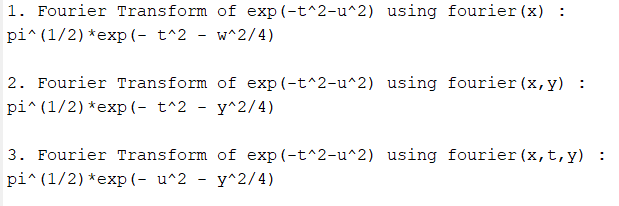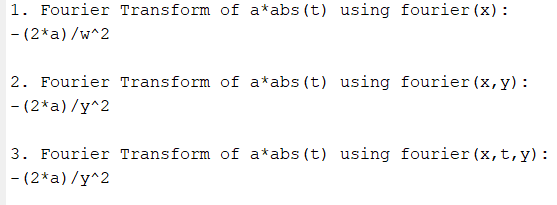# Fourier transform in MATLAB

• Difficulty Level : Medium
• Last Updated : 30 May, 2021

Fourier Transform is a mathematical technique that helps to transform Time Domain function x(t) to Frequency Domain function X(ω). In this article, we will see how to find Fourier Transform in MATLAB.

The mathematical expression for Fourier transform is:Using the above function one can generate a Fourier Transform of any expression. In MATLAB, the Fourier command returns the Fourier transform of a given function. Input can be provided to the Fourier function using 3 different syntaxes.

• Fourier(x): In this method, x is the time domain function whereas the independent variable is determined by symvar and the transformation variable is w by default.
• Fourier(x,transvar): Here, x is the time domain function whereas transvar is the transformation variable instead of w.
• Fourier(x,indepvar,transvar): In this syntax, x is the time domain function whereas indepvar is the independent variable and transvar is the transformation variable instead of symvar and w respectively.

Now we find the Fourier Transform of.

Example 1:

## Matlab

 `% MATLAB code to specify the variable t ``% and u as symbolic ones The syms function``% creates a variable dynamically and ``% automatically assigns to a MATLAB variable``% with the same name``syms t u`` ` `% define time domain function x(t)``x = exp(-t^2-u^2);`` ` `% fourier command to transform into ``% frequency domain function X(w)`` ` `% using 1st syntax, where independent variable``% is determined by symvar (u in this case)``% and transformation variable is w by default.``X = fourier(x);`` ` `% using 2nd syntax, where transformation ``% variable = y``X1=fourier(x,y);`` ` `% using 3rd syntax, where independent ``% variable = t & transformation variable = y ``X2=fourier(x,t,y);`` ` `% Display the output value``disp(``'1. Fourier Transform of exp(-t^2-u^2) using fourier(x) :'``)``disp(X);`` ` `disp(``'2. Fourier Transform of exp(-t^2-u^2) using fourier(x,y) :'``)``disp(X1);`` ` `disp(``'3. Fourier Transform of exp(-t^2-u^2) using fourier(x,t,y) :'``)``disp(X2);`

Output:Let’s take another example to find the Fourier Transform of a*abs(t).

Example 2:

## Matlab

 `% MATLAB code for specify the variable``% a and t as symbolic ones``syms a t`` ` `% define time domain function x(t) ``% where t=independent variable & a=constant``x = a*abs(t);`` ` `% fourier command to transform into frequency``% domain function X(w)``% using 1st syntax``X = fourier(x);`` ` `% using 2nd syntax, where transformation ``% variable = y``X1 = fourier(x,y);`` ` `% using 3rd syntax, where transformation variable``% = y & independent``% variable = t (as t is the only other variable)``X2 = fourier(x,t,y);`` ` `% Display the output value``disp(``'1. Fourier Transform of a*abs(t) using fourier(x):'``)``disp(X);`` ` `disp(``'2. Fourier Transform of a*abs(t) using fourier(x,y):'``)``disp(X1);`` ` `disp(``'3. Fourier Transform of a*abs(t) using fourier(x,t,y):'``)``disp(X2);`

Output:My Personal Notes arrow_drop_up